## Using gnrnd4 on a PC

This page describes the purpose of and the use of a set of routines encompassed in the file gnrnd4.R. The purpose and use are strictly related to the material of this course and in particular, to the coordination of data values on many of the web pages in this course and obtaining the same values in an R session.

There are many times when web pages in this course make reference to a collection of data values. For example, consider the
Now, although the particular web page may wish to talk about this data, it would be even better, and in the case of tests on web pages it may be highly desirable, if you could create a vector of the same values on your computer, and to do so in R.

If the web page is being displayed on a computer, then one possible solution would be to highlight the data in the table, copy it, and paste it to a file. Were we to do that then the file would have values in a number of rows and in a number of columns. R would have a hard time reading that directly, but we could manage it, with a lot of extra work. We would have to find some way to read that into R.

Another solution would be to copy the contents of the table and paste it into some program such as Microsoft Excel, where we could manipulate the data until we could write it to a file in a form that R could read.

Of course, neither of those solutions would be of any help if we were reading Table 1 on paper, as would be the case for a test. The obvious solution would be to just type the values into R. We have already seen the command to do this, namely:

But who wants to type that? In fact, I am sure that I couldn't type that without making at least one error! One interesting note here is that rather than being presented in table format if the values were presented as a comma separated list, such as we just saw in the command above, then we would have an easier time, using Excel, in converting them to a form that we could eventually read into R. But that still does not help in the printed version of the collection of values.

The values given in Table 1 above are generated, each time the page is loaded or refreshed, by a Javascript program that is part of this web page. In fact, if you were to use your browser to look at the page source then you would be able to find and read that Javascript program. The gnrnd4 solution to our challenge is just a function in R that performs exactly the same computations as did the Javascript program. Such a R function is provided in the file gnrnd4.R. The trick is
1. to get that file
2. to load it into R so that you can use it
3. to give the R function the starting point used by the Javascript program to generate the values.
There are a number of ways to achieve points 1 and 2. On my web page there is a listing that gives all of the routines that I have written for this course. It is on the page Table of Links to various R scripts. Part of that table provides a link to eaach of the routines. You could use that link to download the file to your computer and then copy it into any R session that you are using.

A second approach would be to configure a R command that would directly read the file from my web site. The R command to do that is
source("http://courses.wccnet.edu/~palay/math160r/gnrnd4.R")

The third approach, and the one I want us to use, takes into account the fact that the USB drive given out in class actually has the desired file on it. You can have R load the file from that drive. The steps that follow walk you through doing this. Our goal will be to generate the values in the following table:

The eventual command that we will use to generate these same values in our R session is `gnrnd4(key1=364527804,key2=1600123)`. However, we want to do a number of steps to set up all of this.

The work here was done on a PC running Windows 10.

We start by inserting the USB drive. Windows opens File Explorer to give us a view of the contents of the drive. The top of that view is shown in Figure 0.

Figure 0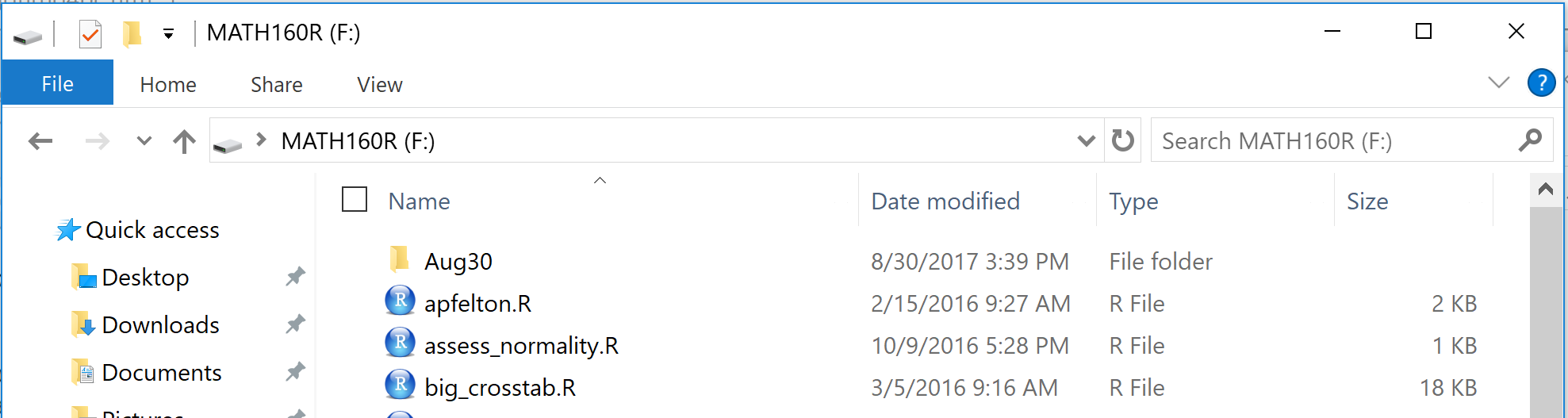If the expanded menu shown in Figure 1 is not visible, just click on the Home menu item, circled in green in Figure 1, to display that expaned menu. Within that menu we want to click on the Few Folder icon as shown in Figure 1.

Figure 1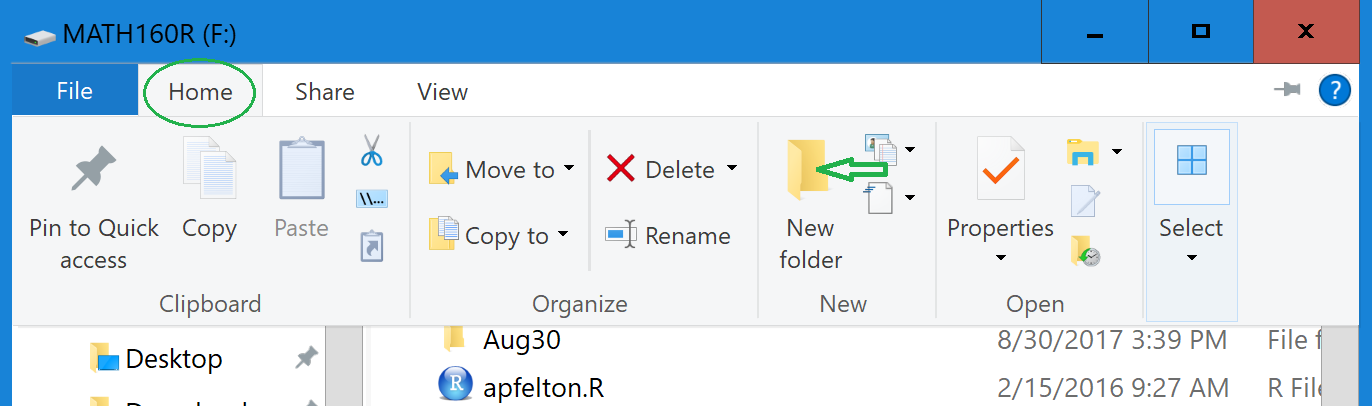This creates a new filder with the default name New folder, a name we would like to change now.

Figure 2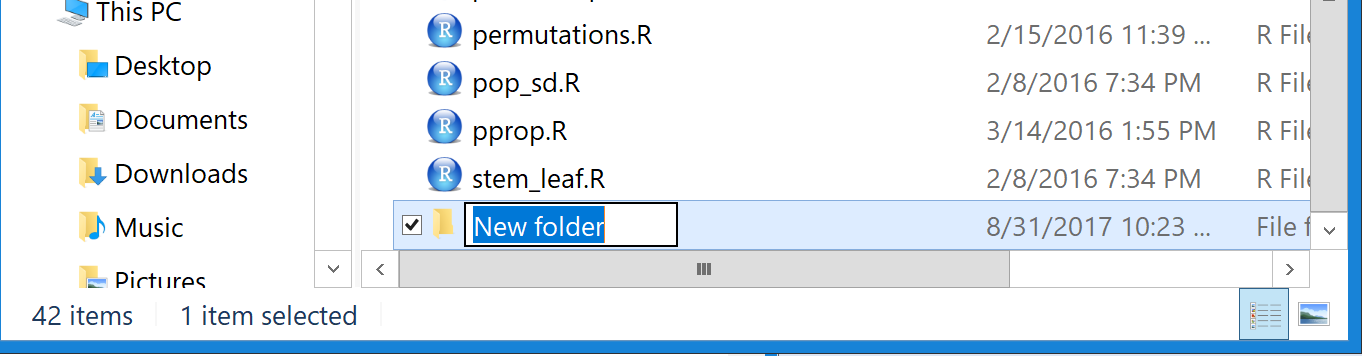Because the name New folder is highlighted in Figure 2 we can just enter our new name; in this eample we will call it project02, as shown in Figure 3.

Figure 3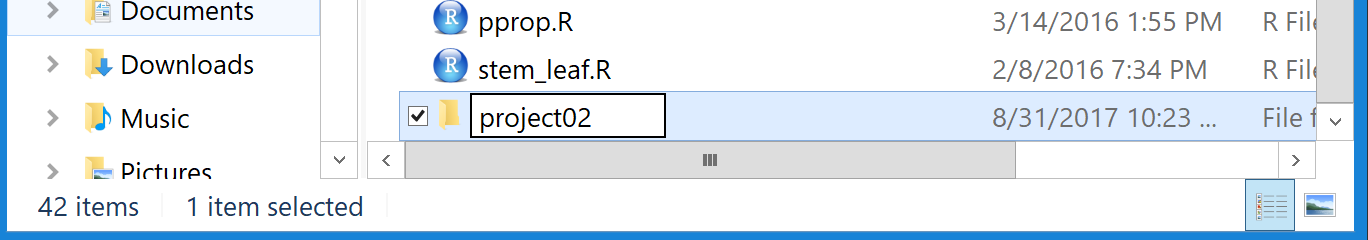Our next step is to get a copy of the file model.R from the top directory (folder) of our USB drive. Figure 3 is still displaying that top directory. Therefore, we point to the file name, model.R, and "right-click" on that file name. This opens an action pane shown in Figure 4.

Figure 4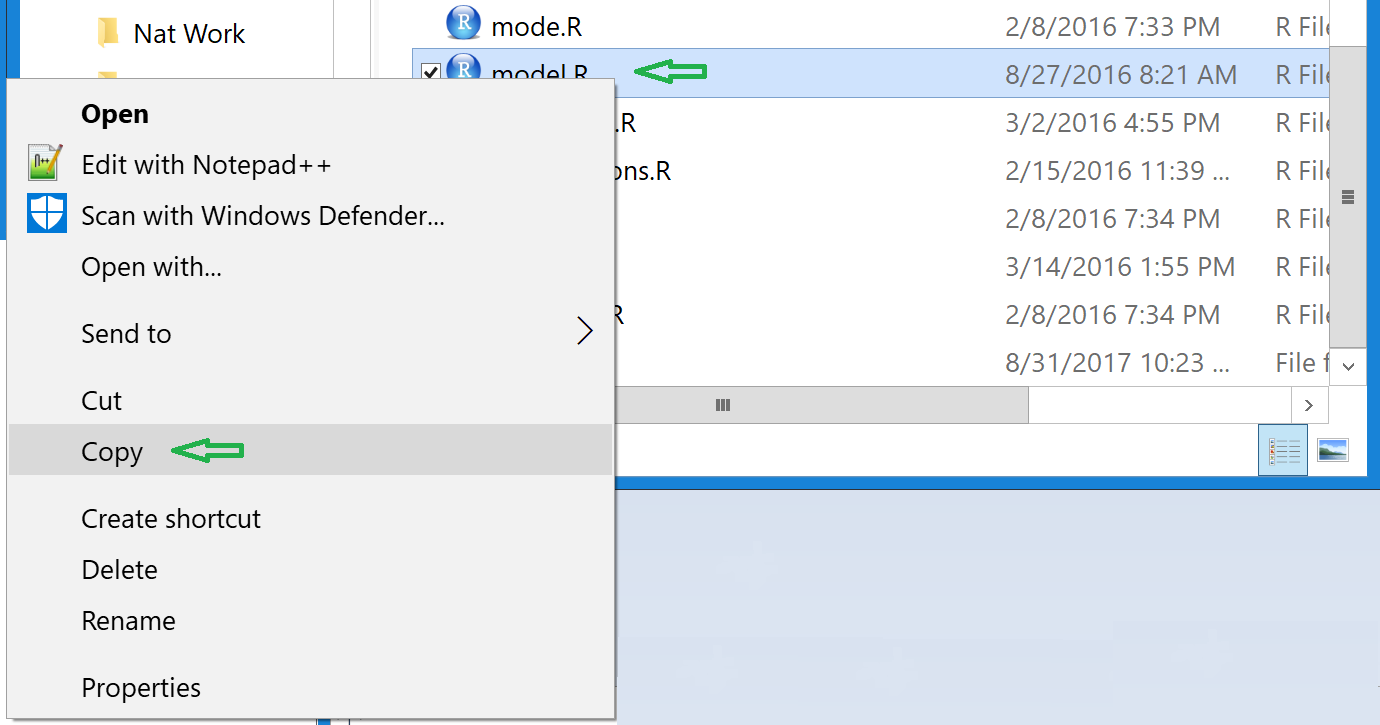Of all the actions shown, the one we want is Copy. To do this we just click on that action.

At this point the computer has a copy of the file. We want to put that copy into our new directory, project02. To do this we need to navigate to that new directory. We do this by doublie clicking on the directory name, as shown in Figure 5.

Figure 5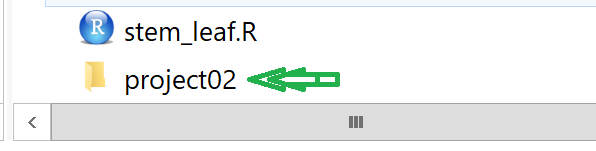This causes File Explorer to display the contents if the project02 foldere, shown in Figure 6.

Figure 6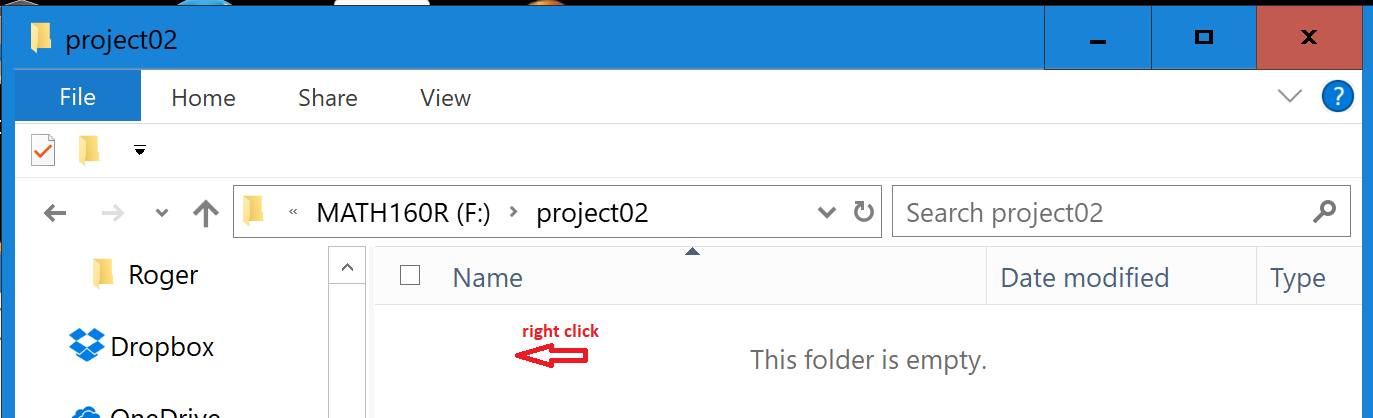As expected, since we just created the folder, it is empty. But not for long. We just right-click in th files area of Figure 6 and this opens a new actions pane, shown in Figure 7.

Figure 7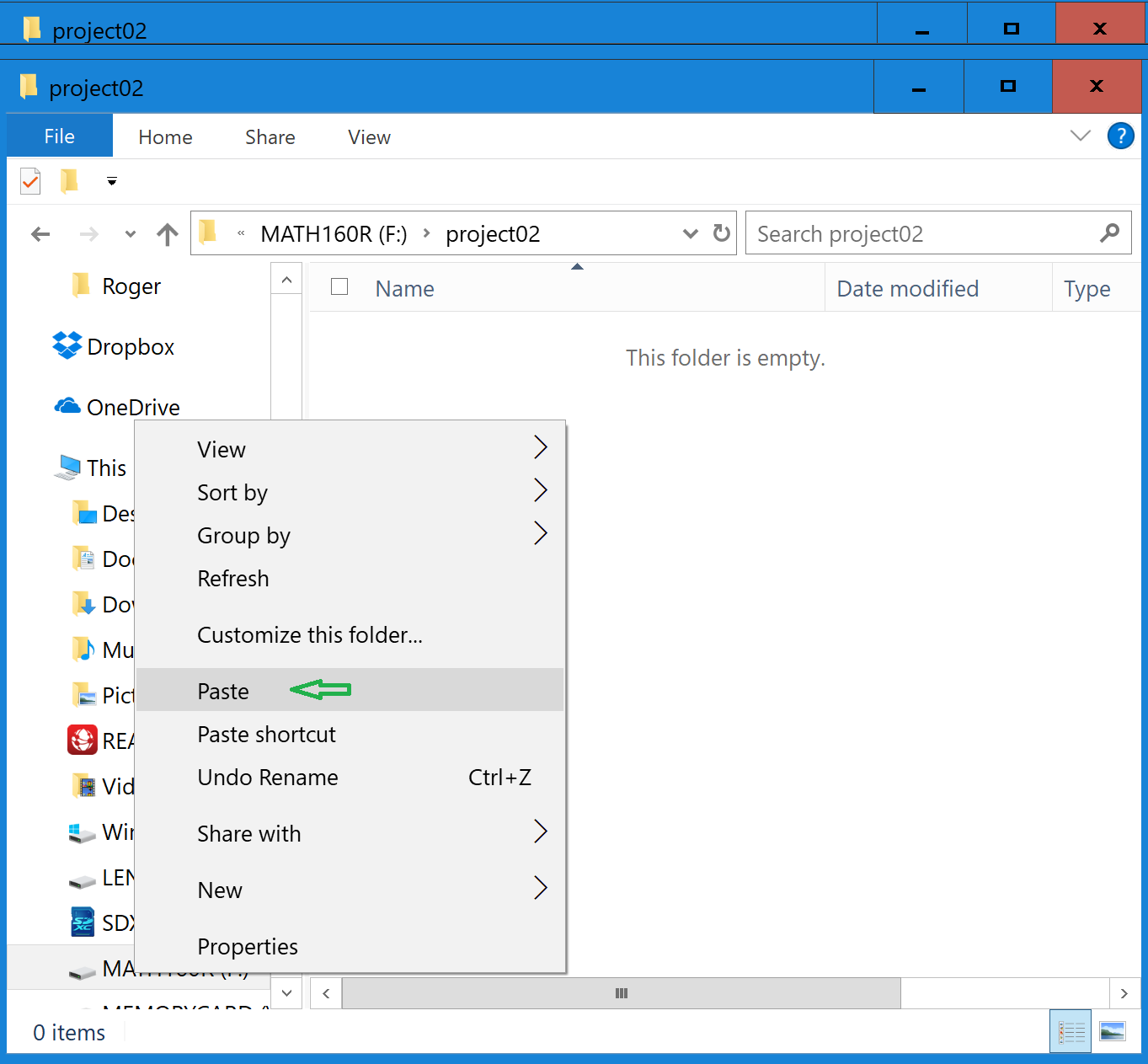There we click on the Paste option. The result, shown in Figure 8, is that we now have a copy of model.R in our new sub-directory, project02.

Figure 8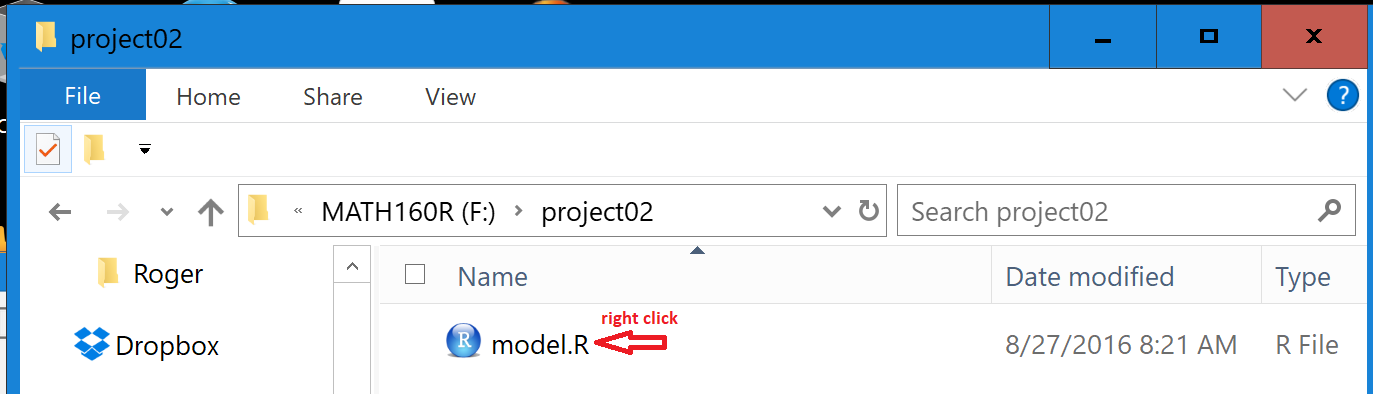Now we want to change the name of the file to something more appropriate. We point to the name in Figure 8 and then right click on it to open the action window shown in Figure 9.

Figure 9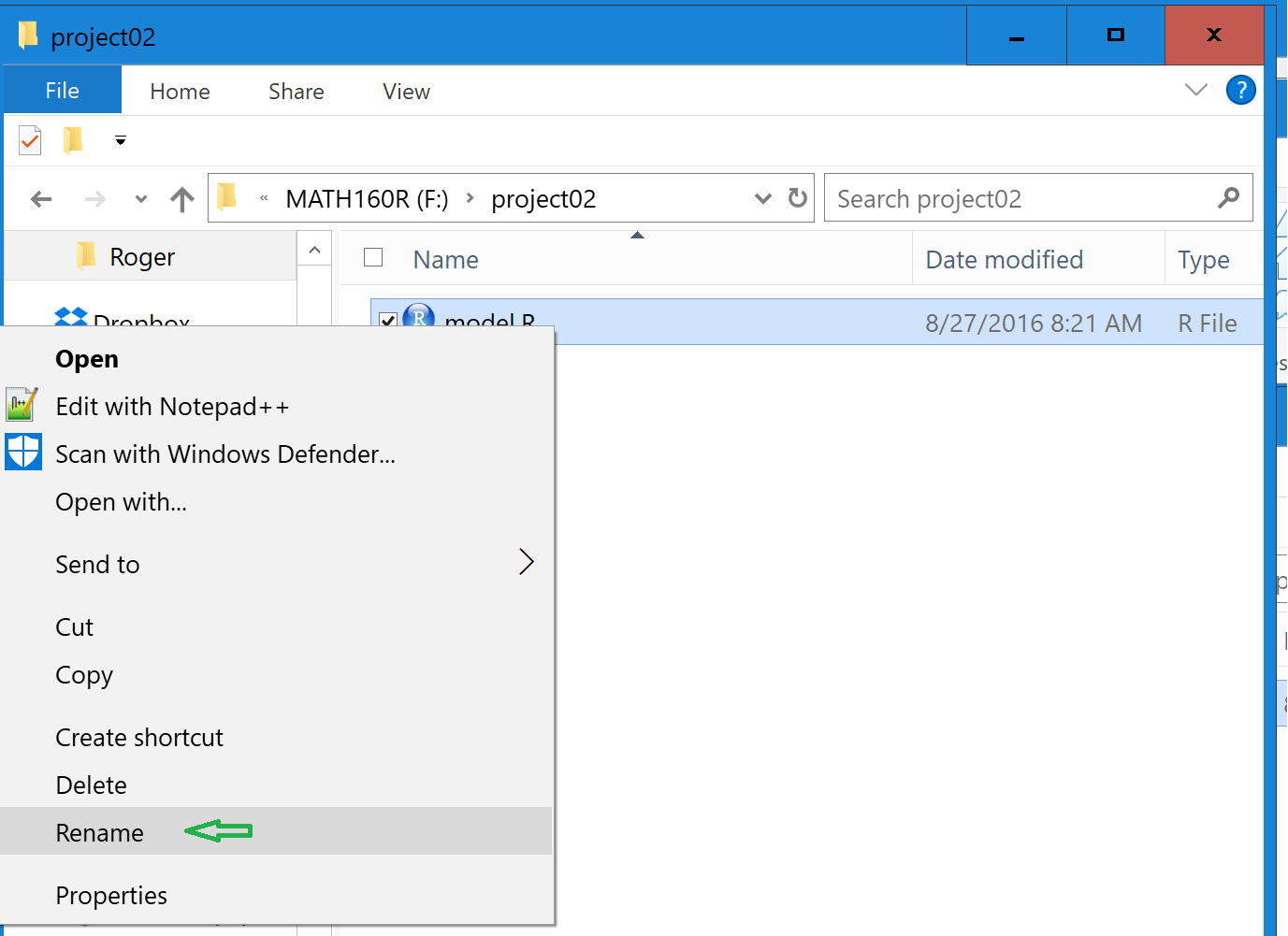Click on the Rename option. This will highlight the file name, as shown in Figure 9a.

Figure 9a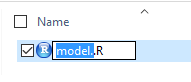Then type in the new name, we will use the name demo_gnrnd4.R and we get Figure 10.

Figure 10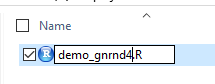Then we double click on that file name, shown in Figure 10a, in order to open our RStudio session.

Figure 10a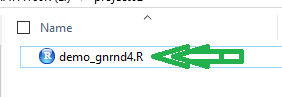Figure 11 shows the entire RStudio window.

Figure 11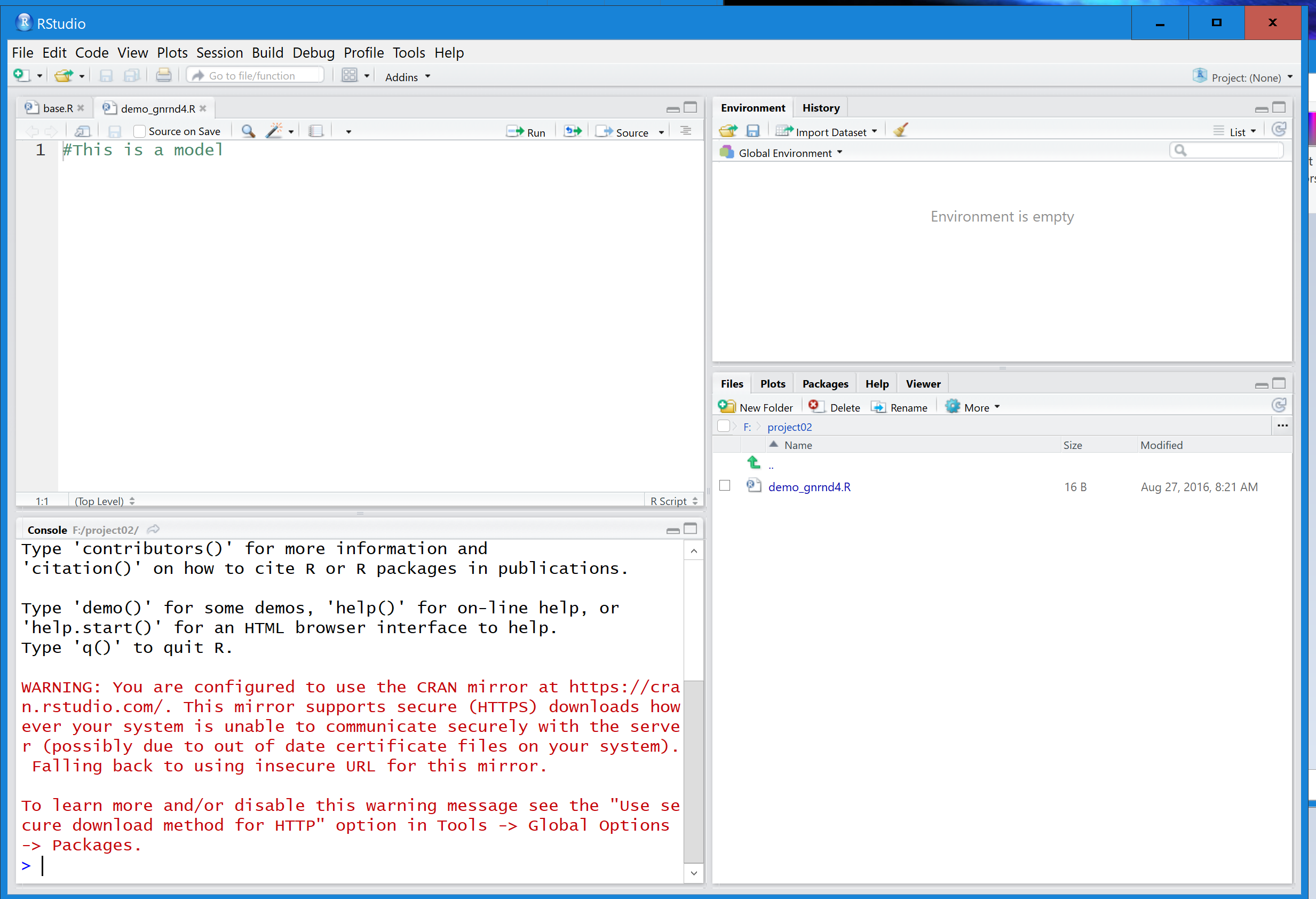If we look, in Figure 12, at the upper left pane, the editor pane, we see that RStudio has opened our new file, demo_gnrnd4.R.

Figure 12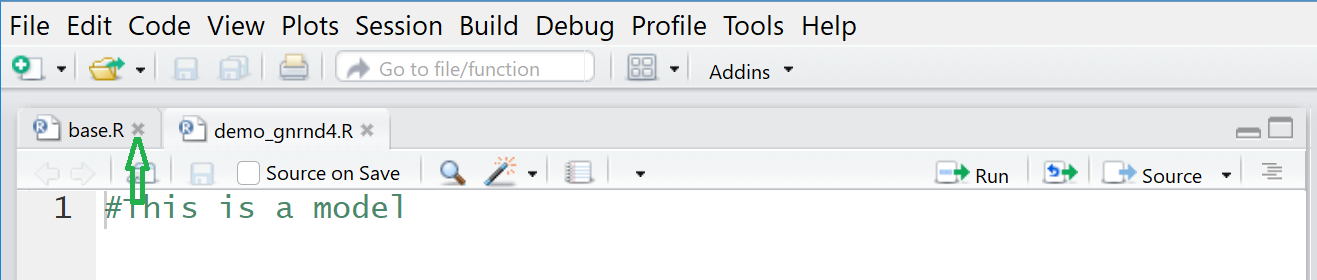Note, in Figure 12, that there is another file, in this instance base.R, that is in this editor. This is left over from an earlier run of RStudio. We do not want this file in this session. Therefore we click on the little "x" after that file name in order to close it. That leaves us with just the demo_gnrnd4.R file in the editor, as shown in Figure 13.

Figure 13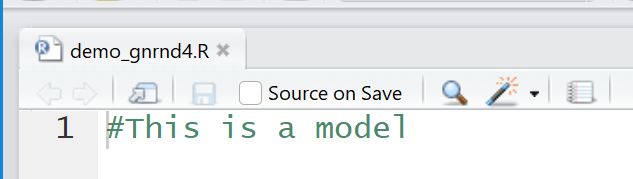If we turn our attention to the lower left pane of Figure 11, we seeFigure 14. IF you are following alon g on your own computer you may not see any of the red warning message. That red warning message is in Figure 14 because I had no internet connection while I was doing this session. Since we have no need for an internet connection in this eample, the warning can be ignored.

Figure 14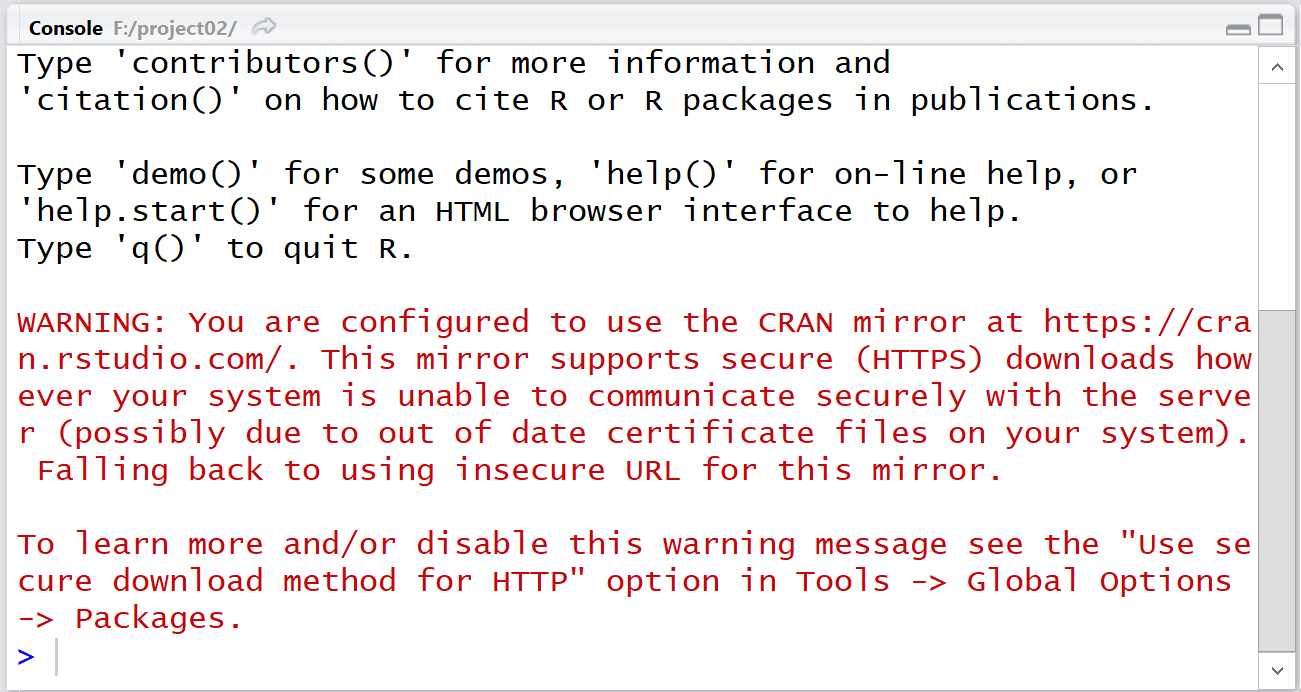The environment pane, shown in Figure 15, is empty. That is a result of our having started in a new folder, project02, without any previous run of R in this folder. We are starting with a clean slate.

Figure 15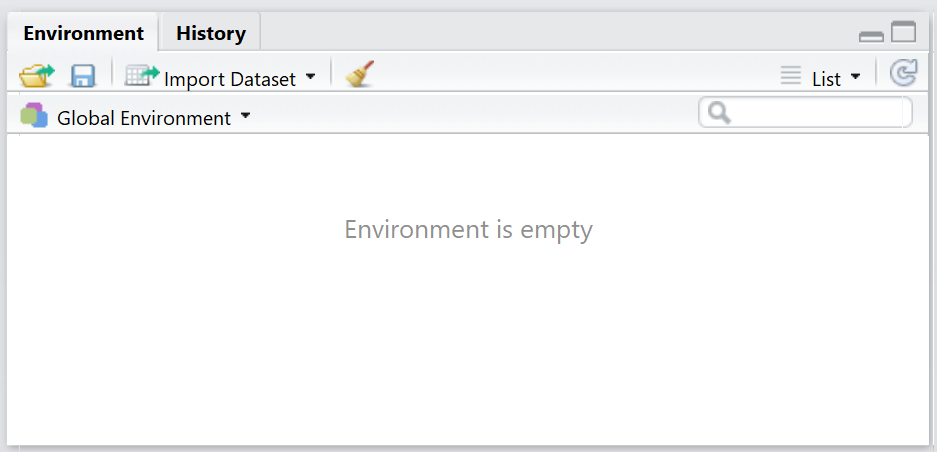Returning to the editor pane, we want to type some new comment lines and then type the one command `source("../gnrnd4.R")` as shown in Figure 16. To make life a bit easier for you, the lines are
```#This is an example of using gnrnd4.
#   I have a web page that tells me that I can generate
#   specific data values in a given table by using
#   the gnrnd4 routine with given arguments.
#   I will try it here.

#  First we need to get the gnrnd4 routine into our
#  current environment.  the routine is on this USB
#  drive, in the directry (folder) directly above
#  this one.  So we use the command

source("../gnrnd4.R")
```
If you are following allon on your own computer you can copy the lines from this web page and just paste them into your RStudio session.

Figure 16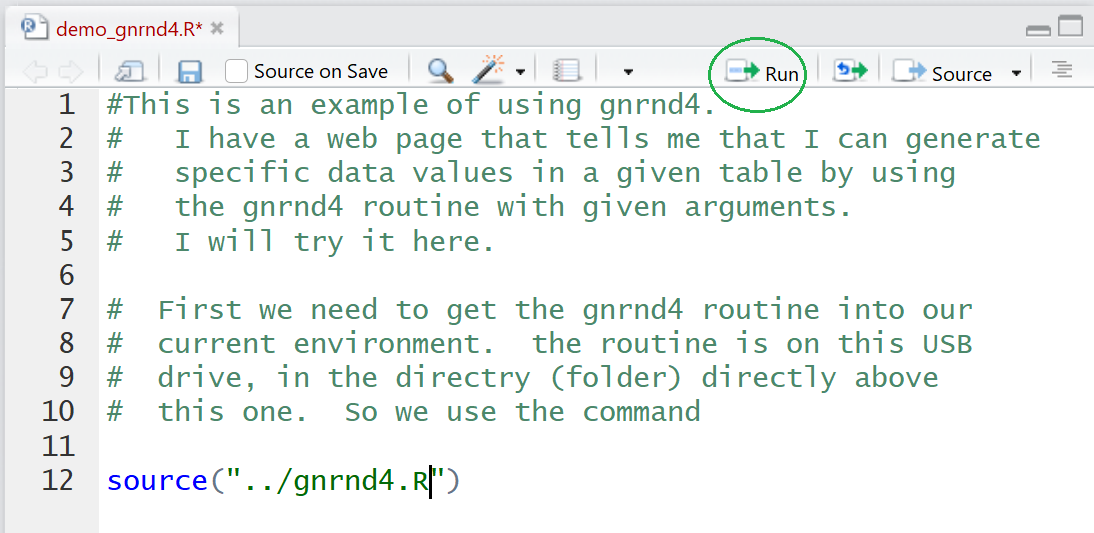Figure 17 shows just lines 11 and 12 of Figure 16. Notice that the cursor is on line 12, the line containing the command that we want to run. To run that command click on the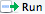at the top of the editor pane, circled back in Figure 16.

Figure 17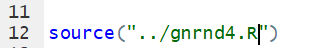The result, shown in the console pane in Figure 17a, is that the command is run in R. It produces no output.

Figure 17a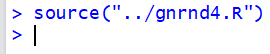However, if we return to the environment pane, Figure 18, we see that two new values and two new functions have been defined.

Figure 18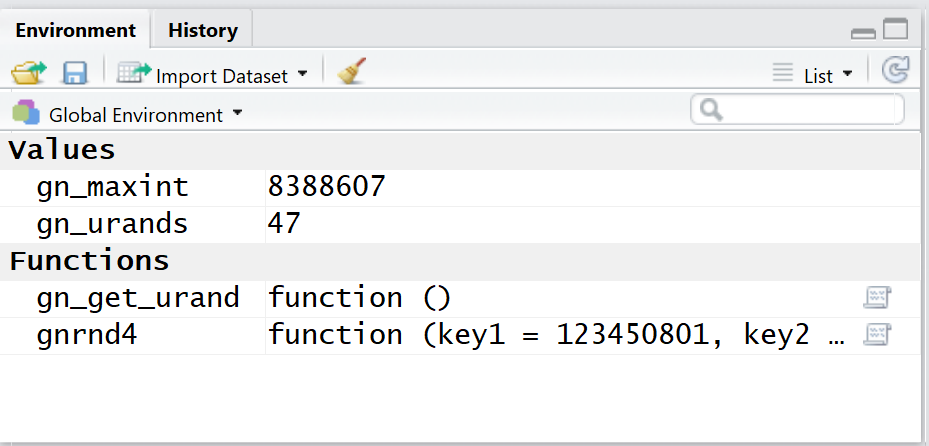Now that the function has been loaded into our environment we can actually use it. Figure 19 shows the new lines that we have added in the editor pane. They are reproduced here so that you can copy them if so desired into your RStudio session.
```
#  once it is loaded we can use it.  The two
#  arguments that we were given were
#  key1=364527804 and key2=1600123.  We use those
# to make the command

gnrnd4(key1=364527804,key2=1600123)
```

Figure 19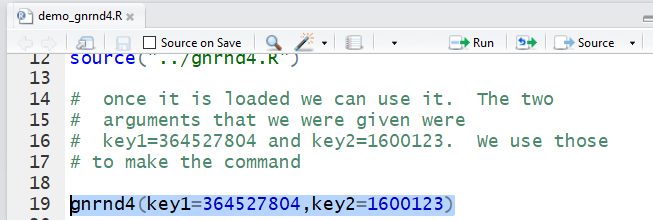In Figure 19 we have hghlighted the command that we actually want to execute. We click on theto actually run that hghlighted command. The result is seen, in the console pane, in Figure 20.

Figure 20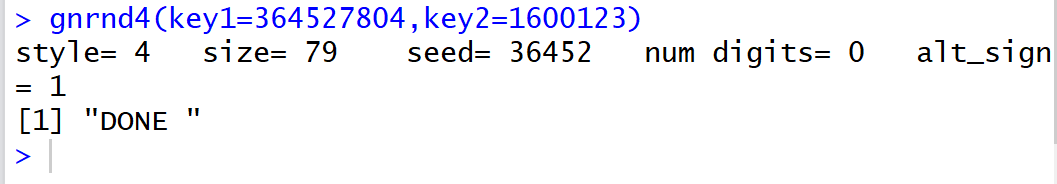This time there was actually some output, but its meaning is not completely clear. On the other hand, if we look back at the environment pane, shown in Figure 21, we see that we now have two new variables, one of which, `L1`, seems to hold the numbers from Table 2 that we were trying to create in our RStudio session.

Figure 21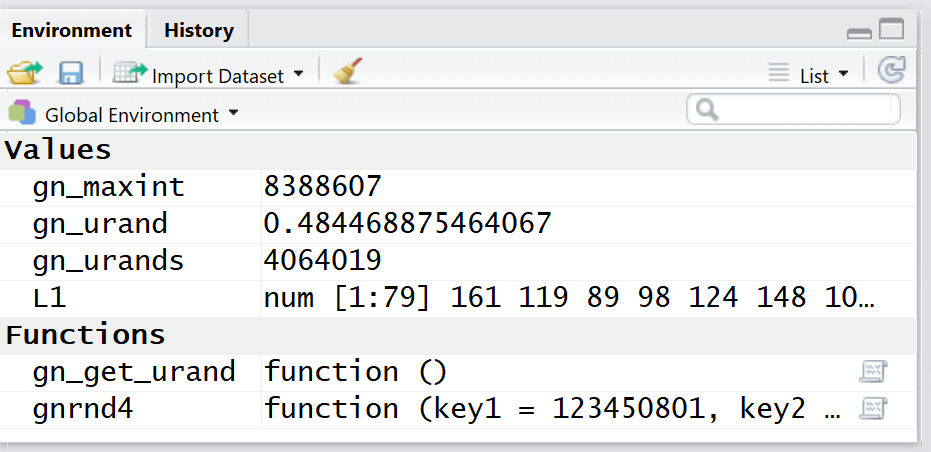If we want to see all of the contents of `L1` we can just use that variable name. As has been our practice we will first add it to the editor pane. That is done in Figure 22.

Figure 22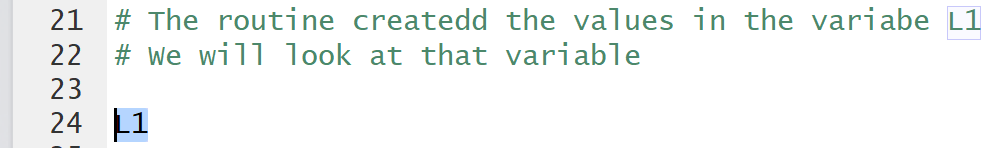Then, using the we have RStudio perform that command in the console pane shown in Figure 23.

Figure 23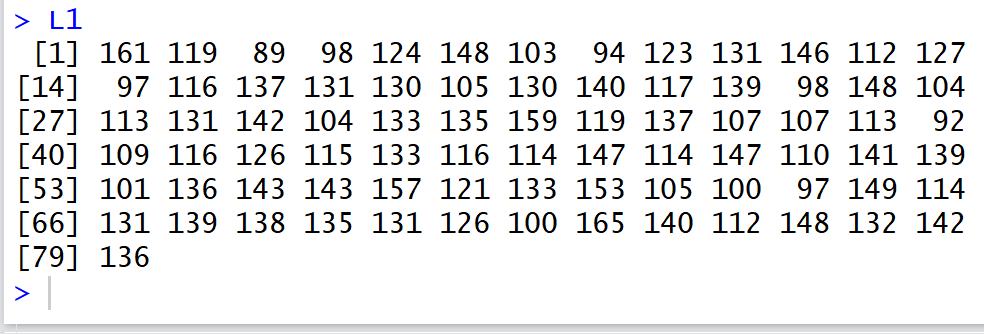At this point we are essentially done. We can give the command `q()` directly in the console pane as shown in Figure 24.

Figure 24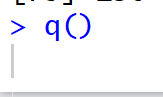When we try to quit the session RStudio recognizes that we have been adding things to the file in the editor, namely, to the file demo_gnrnd4.RRStudio does not want us to accidently lose our work so it gives us the opetion, shown in Figure 25, to save that file.

Figure 25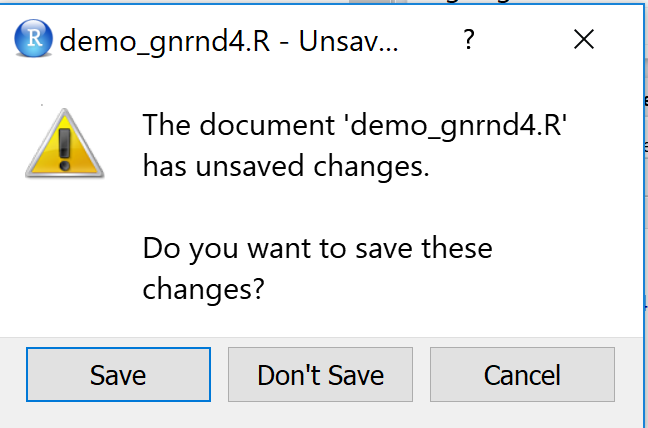Once we click on the Save button in Figure 25 RStudio returns us to the console pane where R asks if we want to save the workspace. We respond in Figure 26 wth a `y`.

Figure 26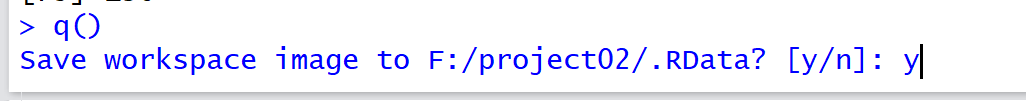Pressing e Enter key concules our RStudio session. this also returns us to the File explorer screen which now shows the current contents of our directory project02.

Figure 27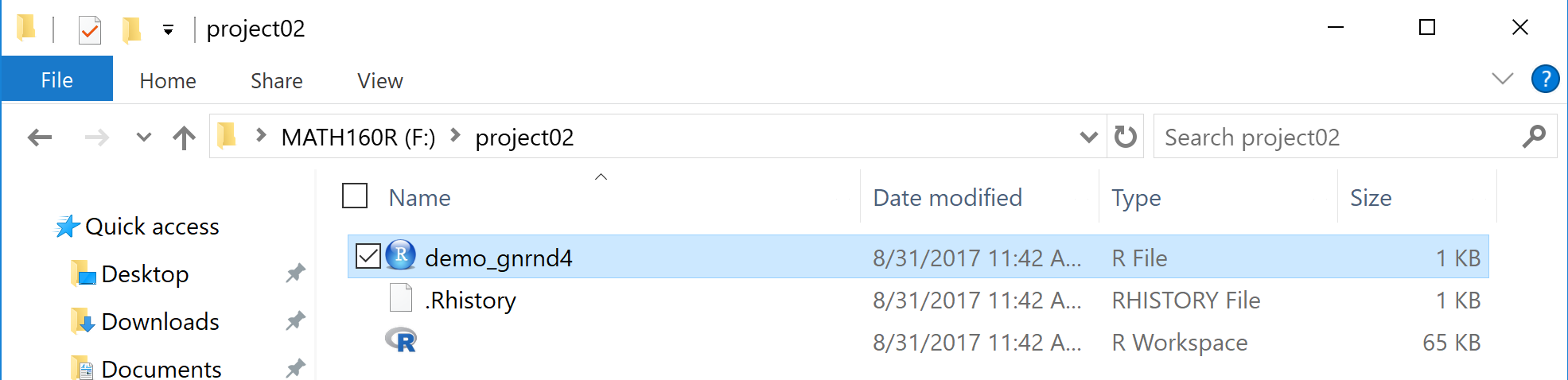The conents of Figure 27 seem a little strange. First of all there are now three files in the directory. The file demo_gnrnd4.R is expected. you might note that it has now grown to be `1KB` long. The other two files remain a bit of a mystery with the last one showing up as and icon but without even a name. This last issue is caused, in this case, because the computer on which this was run did not have the File name extensions switch turned on.

We can fix that problem by first clicking on the View menu option. That will display the action menu shown in Figure 28.

Figure 28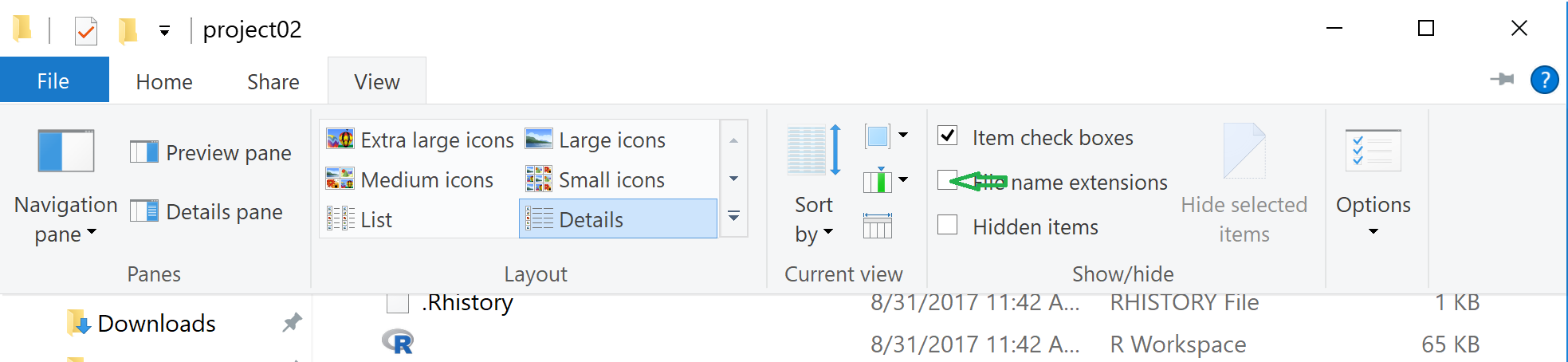Click on the box before File name extensions and the display changes to that of Figure 29.

Figure 29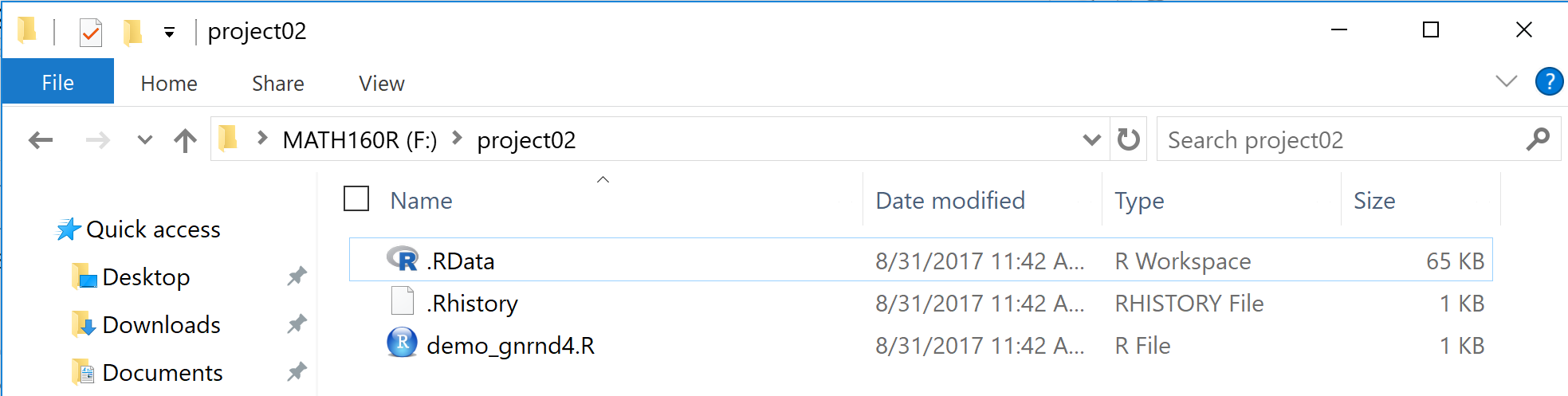Now we see that the two new files are .Rhstory and .RData. OF those two, .RData holds the variables and functions that we had in the environment back in Figure 26 when we closed our session.

If we now double click on the demo_gnrnd4.R file, we will start a new RStudio session, but this time it will start with the environment the way we left it back in Figure 26. You can see this in Figure 30.

Figure 30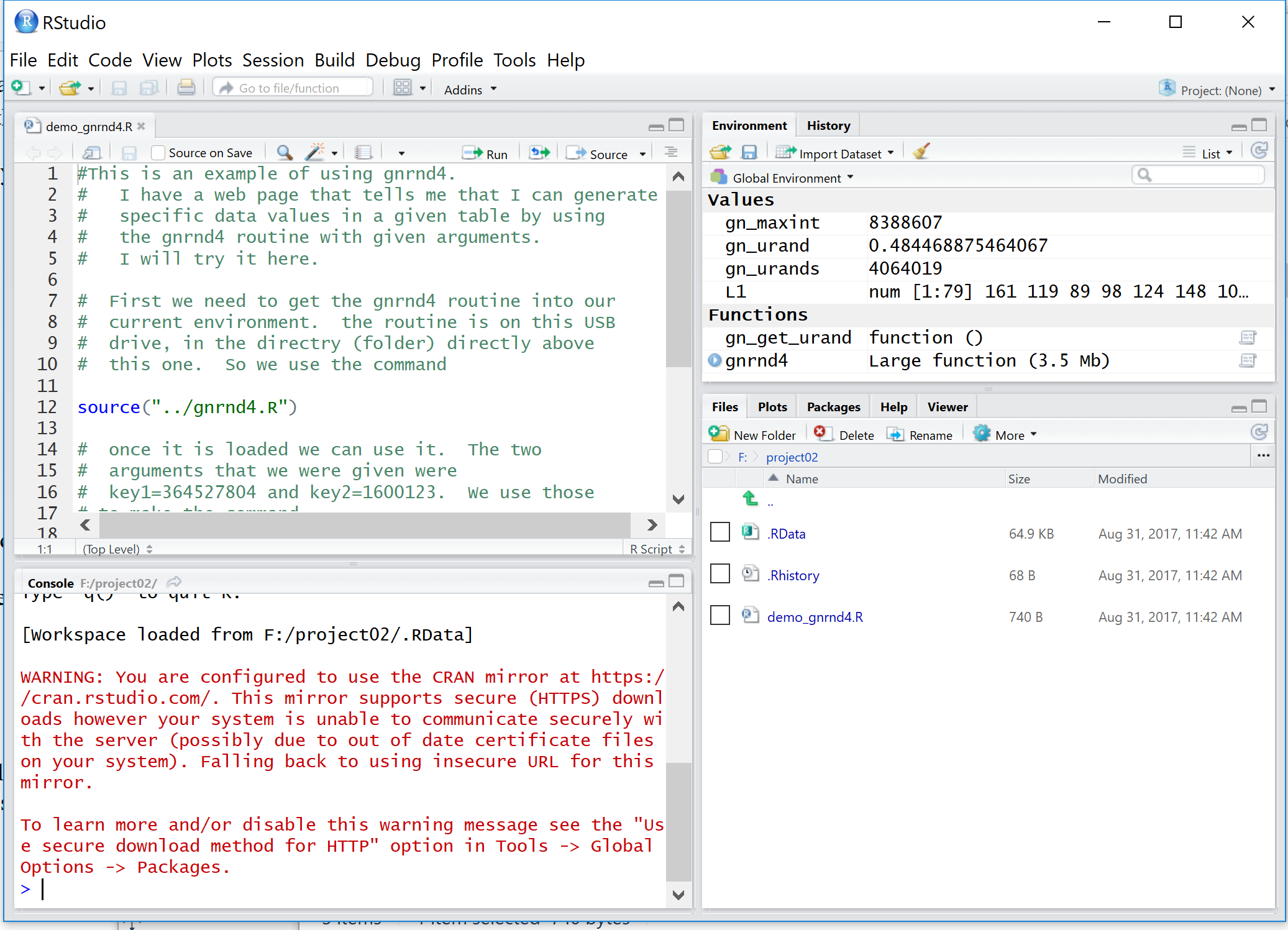We close our RStudio session with the q() command.
```#This is an example of using gnrnd4.
#   I have a web page that tells me that I can generate
#   specific data values in a given table by using
#   the gnrnd4 routine with given arguments.
#   I will try it here.

#  First we need to get the gnrnd4 routine into our
#  current environment.  the routine is on this USB
#  drive, in the directry (folder) directly above
#  this one.  So we use the command

source("../gnrnd4.R")

#  once it is loaded we can use it.  The two
#  arguments that we were given were
#  key1=364527804 and key2=1600123.  We use those
# to make the command

gnrnd4(key1=364527804,key2=1600123)

# The routine createdd the values in the variabe L1
# We will look at that variable

L1

```